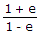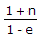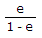# Civil Engineering - Soil Mechanics and Foundation Engineering

### Exercise :: Soil Mechanics and Foundation Engineering - Section 1

46.

The relationship between void ratio (e) and porosity ratio (n) is :

 A. n =B. e =C. n =D. e = n/(1 - n)

Explanation:

No answer description available for this question. Let us discuss.

47.

Failure of the stability of slopes, generally occurs along

 A. slip plane B. a horizontal surface C. a curved surface D. all the surfaces.

Explanation:

No answer description available for this question. Let us discuss.

48.

Pick up the correct statement from the following:

 A. Failure plane carries maximum shear stress B. Failure plane does not carry maximum shear stress C. Failure plane carries shear stress equal to maximum shear stress D. None of these.

Explanation:

No answer description available for this question. Let us discuss.

49.

Geologic cycle for the formation of soil, is

 A. Upheavel → transportation → deposition → weathering B. Weathering → upheaval → transportation → deposition C. Transportation → upheaval → weathering → deposition D. Weathering → transportation → deposition → upheaval E. None of these.

Explanation:

No answer description available for this question. Let us discuss.

50.

The weight of a pycnometer containing 400 g sand and water full to the top is 2150 g. The weight of pycnometer full of clean water is 1950 g. If specific gravity of the soil is 2.5, the water content is

 A. 5% B. 10% C. 15% D. 20%